# CBSE Class 11 Physics Chapter 9 Revision Notes

Chapter 9: Mechanical Properties Of Solids Revision Notes

• Inter molecular Forces: In a solid, atoms and molecules are arranged in such a way that the forces exerted by neighboring molecules act on each molecule. Inter molecular forces are the name for these forces.
• Elasticity: Elasticity is the ability of a body to return to its original configuration (length, volume, or shape) when deforming forces are removed.
• The forces between a body’s atoms or molecules determine how it changes shape or size when external forces act on it.
• Elastic forces are atomic forces that have a short range.
• Perfectly Elastic Body: A perfectly elastic body is one that returns to its original configuration immediately and completely after a deforming force is removed from it. Quartz and phosphor bronze are two examples of nearly elastic materials.
• Plasticity is the inability of a body to return to its original size and shape even after the deforming force has been removed, and such a body is referred to as a plastic body.
• Stress: The ratio of the internal force F produced when the substance is deformed to the area A over which this force acts is defined as stress. This force is equal in magnitude to the externally applied force when it is in equilibrium.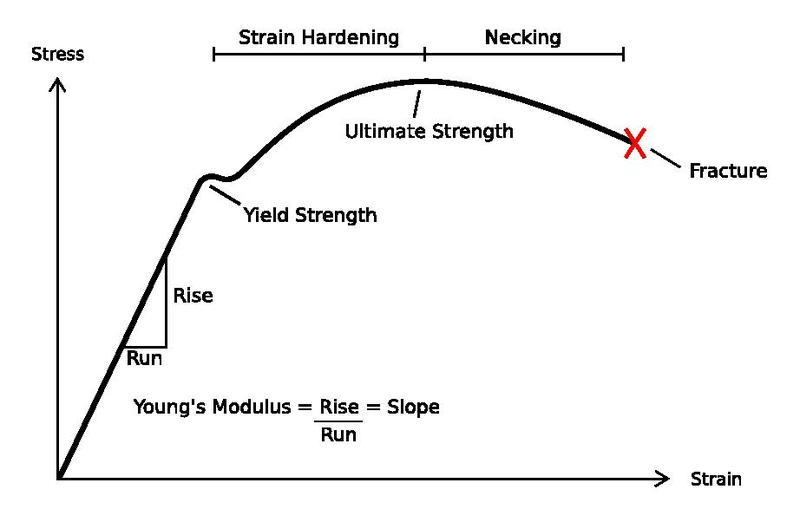Source

• There are two types of stress:

(i) Normal stress: The restoring force per unit area perpendicular to the body’s surface is defined as normal stress. Tensile and compressive stress are the two types of normal stress.

(ii) Tangential stress: Tangential stress occurs when the elastic restoring force or deforming force acts parallel to the surface area.

Strain: The ratio of the change in size or shape to the original size or shape is what it’s called. It is just a number with no dimensions.

• There are three types of strains:

(i) Longitudinal strain: The strain produced in the body is called longitudinal strain or tensile strain if the deforming force produces only a change in length. It is written as follows: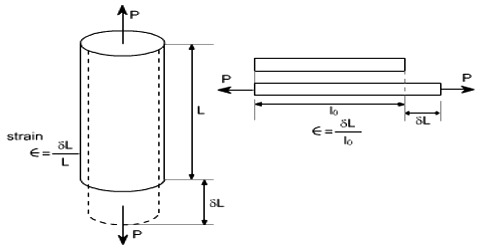Source

(ii) Volumetric strain: When a deforming force causes only a change in volume, the strain in the body is referred to as volumetric strain.

(iii) Shear strain: Shear strain is the angle tilt in the body caused by tangential stress expressed. It is written as follows:Source

• Hooke’s Law: Hooke’s law states that within elastic limits, the ratio of stress to the corresponding strain produced is constant. The modulus of elasticity is the name given to this constant.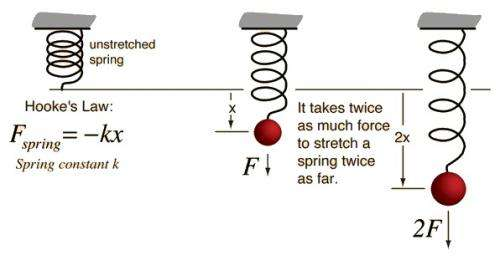Source

• Stress Strain Curve: Stress-strain curves are useful for determining a material’s tensile strength. A stress-strain curve for a given metal is depicted in the diagram.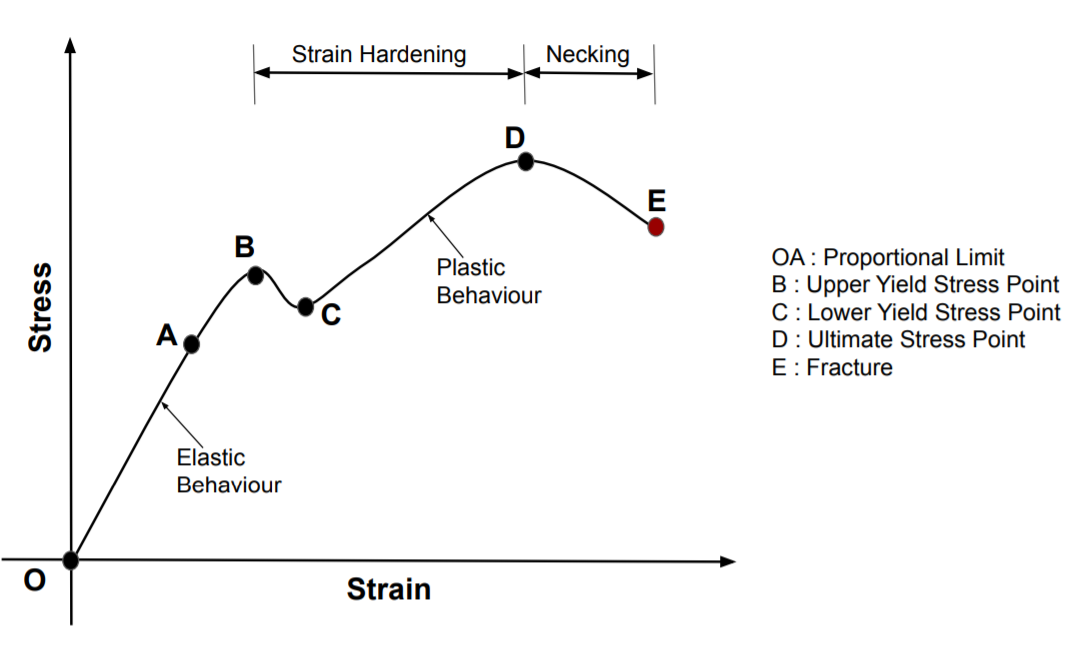Source

• Young’s Modulus: Young’s modulus of elasticity within the elastic limit is defined as the ratio of longitudinal stress to longitudinal strain for a solid in the form of a wire or a thin rod. It is written as follows: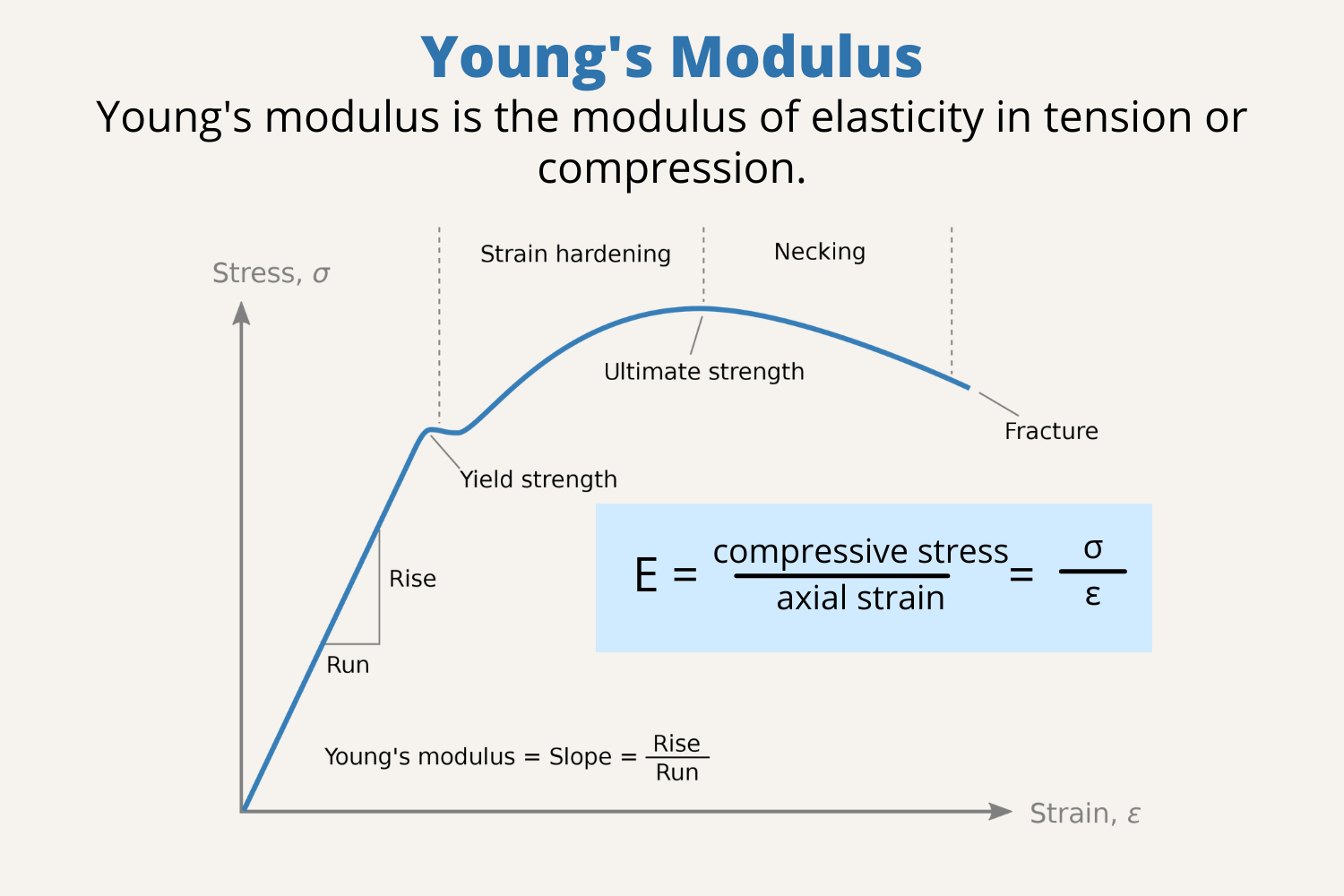Source

• Bulk Modulus: The bulk modulus is defined as the ratio of longitudinal stress to volumetric strain within the elastic limit. It is written as follows: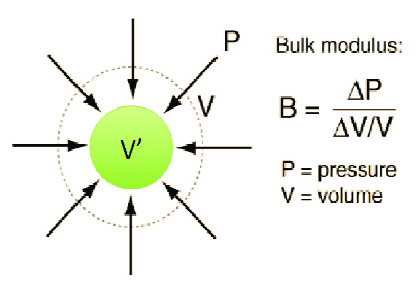Source

• The term “compressibility” refers to the reciprocal of bulk modulus. The fractional change in volume per unit change in pressure is what it’s called.
• Rigidity Modulus or Shear Modulus: It is defined as the tangential stress divided by the shear strain.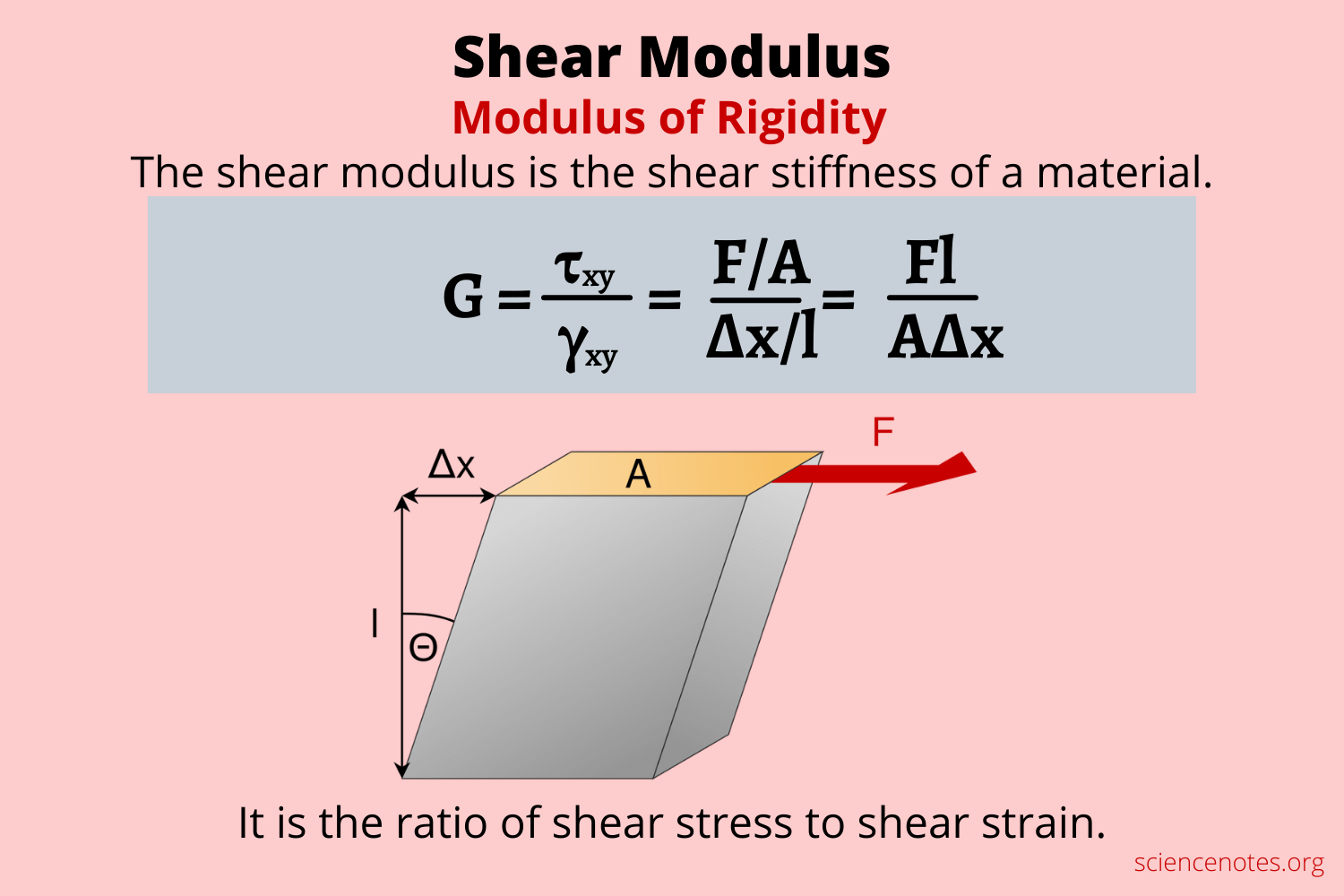Source

• Poisson’s Ratio:
• Lateral strain is defined as the ratio of the change in diameter (D) to the original diameter (D).
• Longitudinal strain is defined as the ratio of the change in length (l) to the original length (l).
• Poisson’s ratio is the ratio of lateral strain to longitudinal strain.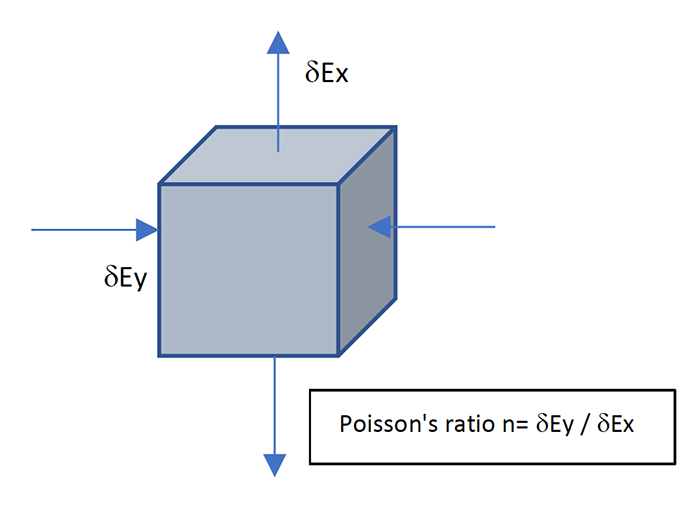Source

]]>